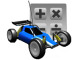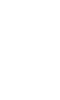#Simple LED Calculator

This calulator will help you figure out the proper resistor(s) needed to safely hook up one or more LEDs to power supplies of various values.

Enter the LED layout, number of LEDs, supply voltage,the Vf, and If LED values. The Vf and If values are found on the LED packaging. Press the button and the schematic will appear showing how to arrange the components. The resistance value will also be calculated.

 LED layout (?): Building List... Number of LEDs: Building List... Supply voltage (?): Building List... LED Vf (forward voltage) (?): Volts LED If (forward current) (?): milliAmpsResistor value (?): Resistor power rating (?): Total current draw (?):

### LED Calculator Using Constant Current Circuit

This calulator will help you figure out the proper resistor needed to safely hook up one or more LEDs to power supplies of various values. Even though this version is slightly more complex, it provides better control over the current no matter what charge state the supply is.

Enter the LED layout, number of LEDs, supply voltage,the Vf, and If LED values. The Vf and If values are found on the LED packaging. Press the button and the schematic will appear showing how to arrange the components. The resistance value will also be calculated.

 LED layout (?): Building List... Number of LEDs: Building List... Supply voltage (?): Building List... LED Vf (forward voltage) (?): Volts LED If (forward current) (?): milliAmpsResistor value (?): Resistor power rating (?): Total current draw (?): LM317T Power Dissupation (?):

##### Other Useful LED Information
• LED Wiring
• LED Formulas
• Using a Linear Voltage Regulator